profileApply-methods {aqp} R Documentation

## Apply a function to soil profiles within a SoilProfileCollection object.

### Description

Apply a function to soil profiles within a SoilProfileCollection object, each iteration has access to a SoilProfileCollection object.

### Usage

```# method for SoilProfileCollection objects
profileApply(object, FUN, simplify=TRUE, ...)
```

### Arguments

 `object` a SoilProfileCollection `FUN` a function to be applied to each profile within the collection `simplify` logical, should the result be simplified to a vector? see examples `...` further arguments passsed to FUN

### Value

When simplify is TRUE, a vector of length `nrow(object)` (horizon data) or of length `length(object)` (site data). When simplify is FALSE, a list is returned.

### Methods

`signature(object = "SoilProfileCollection")`

`slab`, `estimateSoilDepth`

### Examples

``````data(sp1)
depths(sp1) <- id ~ top + bottom

# estimate soil depth using horizon designations
profileApply(sp1, estimateSoilDepth, name='name', top='top', bottom='bottom')
``````
``````## P001 P002 P003 P004 P005 P006 P007 P008 P009
##   89   59   67   62   68  200  233  200  240
``````
``````# scale properties within each profile
# scaled = (x - mean(x)) / sd(x)
sp1\$d <- profileApply(sp1, FUN=function(x) round(scale(x\$prop), 2))
plot(sp1, name='d')
``````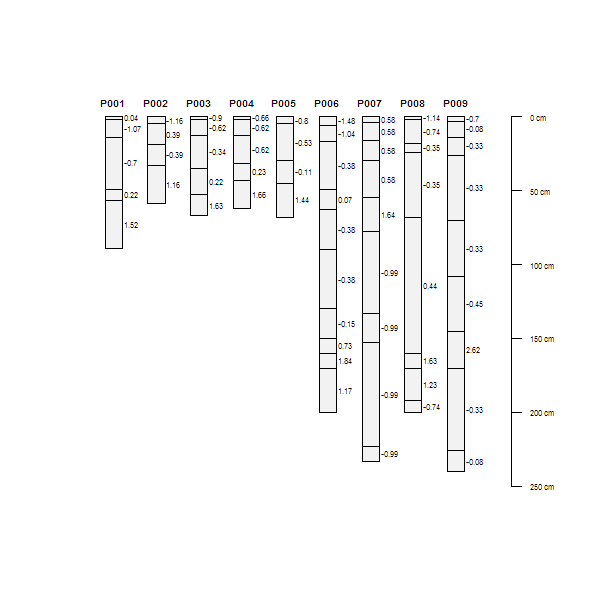``````# compute depth-wise differencing by profile
# note that our function expects that the column 'prop' exists
f <- function(x) { c(x\$prop, diff(x\$prop)) }
sp1\$d <- profileApply(sp1, FUN=f)
plot(sp1, name='d')
``````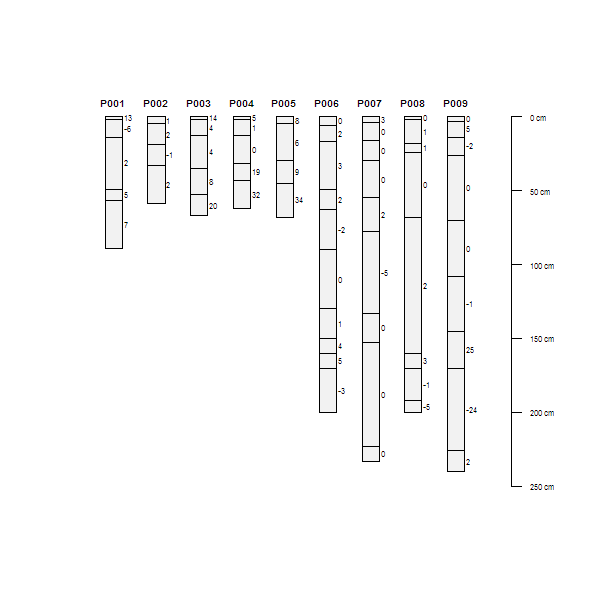``````# compute depth-wise cumulative sum by profile
# note the use of an anonymous function
sp1\$d <- profileApply(sp1, FUN=function(x) cumsum(x\$prop))
plot(sp1, name='d')
``````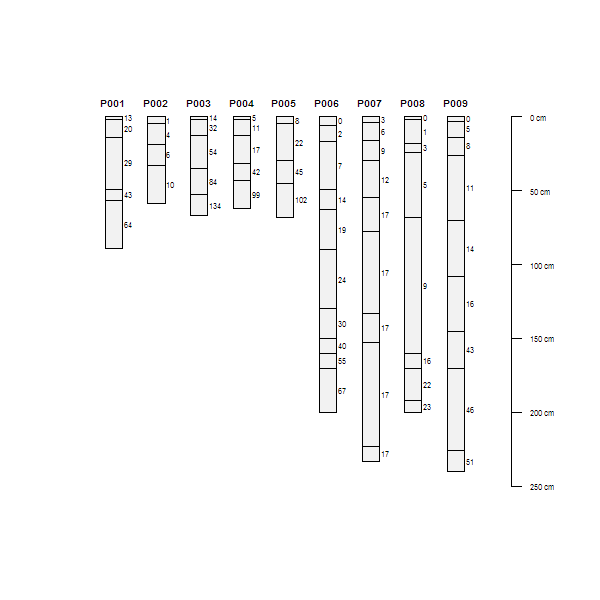``````# compute profile-means, and save to @site
# there must be some data in @site for this to work
site(sp1) <- ~ group
sp1\$mean_prop <- profileApply(sp1, FUN=function(x) mean(x\$prop, na.rm=TRUE))

# re-plot using ranks defined by computed summaries (in @site)
plot(sp1, plot.order=rank(sp1\$mean_prop))
``````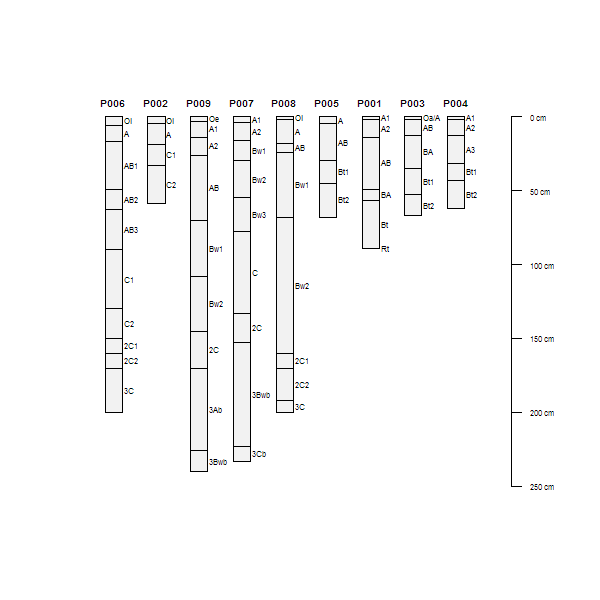``````## iterate over profiles, subsetting horizon data

# example data
data(sp1)

# promote to SoilProfileCollection
depths(sp1) <- id ~ top + bottom
site(sp1) <- ~ group

# make some fake site data related to a depth of some importance
sp1\$dep <- profileApply(sp1, function(i) {round(rnorm(n=1, mean=mean(i\$top)))})

# custom function for subsetting horizon data, by profile
# keep horizons with lower boundary < site-level attribute 'dep'
fun <- function(i) {
# extract horizons
h <- horizons(i)
# make an expression to subset horizons
exp <- paste('bottom < ', i\$dep, sep='')
# subset horizons, and write-back into current SPC
horizons(i) <- subset(h, subset=eval(parse(text=exp)))
# return modified SPC
return(i)
}

# list of modified SoilProfileCollection objects
l <- profileApply(sp1, fun, simplify=FALSE)

# re-combine list of SoilProfileCollection objects into a single SoilProfileCollection
sp1.sub <- do.call(rbind, l)

# graphically check
par(mfrow=c(2,1), mar=c(0,0,1,0))
plot(sp1)
points(1:length(sp1), sp1\$dep, col='red', pch=7)
plot(sp1.sub)
``````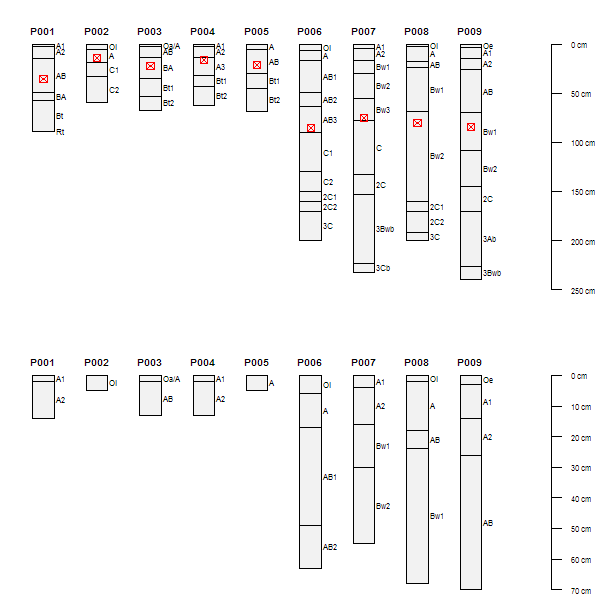``````## Not run:
##D ##
##D ## helper functions: these must be modified to suit your own data
##D ##
##D
##D # compute the weighted-mean of some property within a given diagnostic horizon
##D # note that this requires conditional eval of data that may contain NA
##D # see ?slab for details on the syntax
##D # note that function expects certain columns within 'x'
##D f.diag.wt.prop <- function(x, d.hz, prop) {
##D   # extract diagnostic horizon data
##D   d <- diagnostic_hz(x)
##D   # subset to the requested diagnostic hz
##D   d <- d[d\$diag_kind == d.hz, ]
##D   # if missing return NA
##D   if(nrow(d) == 0)
##D     return(NA)
##D
##D   # extract depths and check for missing
##D   sv <- c(d\$featdept, d\$featdepb)
##D   if(any(is.na(sv)))
##D     return(NA)
##D
##D   # create formula from named property
##D   fm <- as.formula(paste('~', prop))
##D   # return just the (weighted) mean, accessed from @horizons
##D   s <- slab(x, fm, slab.structure=sv, slab.fun=mean)\$value
##D   return(s)
##D }
##D
##D
##D # conditional eval of thickness of some diagnostic feature or horizon
##D # will return a vector of length(x), you can save to @site
##D f.diag.thickness <- function(x, d.hz) {
##D   # extract diagnostic horizon data
##D   d <- diagnostic_hz(x)
##D   # subset to the requested diagnostic hz
##D   d <- d[d\$diag_kind == d.hz, ]
##D   # if missing return NA
##D   if(nrow(d) == 0)
##D     return(NA)
##D
##D   # compute thickness
##D   thick <- d\$featdepb - d\$featdept
##D   return(thick)
##D }
##D
##D
##D # conditional eval of property within particle size control section
##D # makes assumptions about the SPC that is passed-in
##D f.psc.prop <- function(x, prop) {
##D   # these are accessed from @site
##D   sv <- c(x\$psctopdepth, x\$pscbotdepth)
##D   # test for missing PCS data
##D   if(any(is.na(sv)))
##D     return(NA)
##D
##D   # this should never happen... unless someone made a mistake
##D   # check to make sure that the lower PSC boundary is shallower than the depth
##D   if(sv > max(x))
##D     return(NA)
##D
##D   # create formula from named property
##D   fm <- as.formula(paste('~', prop))
##D   # return just the (weighted) mean, accessed from @horizons
##D   s <- slab(x, fm, slab.structure=sv, slab.fun=mean)\$value
##D   return(s)
##D }
##D
##D # try with some sample data
##D data(loafercreek, package='soilDB')
##D
##D profileApply(loafercreek, f.diag.wt.prop, d.hz='argillic horizon', prop='clay')
##D profileApply(loafercreek, f.diag.thickness, d.hz='argillic horizon')
##D profileApply(loafercreek, f.psc.prop, prop='clay')
##D
## End(Not run)
``````

[Package aqp version 1.9.1 Index]